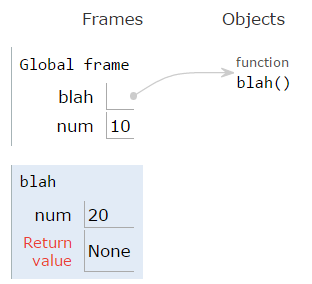# Lesson 04-04: Variable Scope¶

Learning Target: I can understand the scope of a variable.
Learning Target: I can reference global variables within functions.

Whenever we create a variable in python, it’s important to know where it (the variable) can be accessed from - this is called the variable scope. At a basic level, there are two different types of variable scope that we’ll be working with: local and global.

## Local Variables¶

When a variable is local, it means that it can only be accessed from the function that it’s in.

The rule for local variables are: variables within a function can only be accessed from within that function. Check out the following example:

You get an error telling you that the variable num isn’t defined! However, if we consider what appears to be happening in this script, we’re defining a function that sets num to 20. Then we call that function, creating a variable called num and setting it to 20. Our function then ends, returning nothing, and we print out the value of num.

But since num only exists within the function blah, we can’t access it from outside of blah!

Here’s another example:

Here, we don’t come across any error, but it’s clear that the output is not what we might expect. After defining the same function as above, the code appears to be creating a variable num, calling blah() (which should set num to 20), and then printing num - but we see that 10 gets printed!

This is because the num that exists in the function blah is not the same as the num that exists in the main part of the program. We can observe this by looking at the codelens of the program.You’ll notice that both the Global Frame and blah have separate values for num. The num that gets set to 20 only exists in blah, and doesn’t have any affect on the num in the Global Frame.

Arguments in a function are always local. So in the following example:

 1 2 3 4 5 6 7 def add3(a,b,c): return a + b + c x = 1 y = 2 z = 3 print(add3(x,y,z)) 

The variables a, b, and c are local only to the add3 function.

## Global Variables¶

So what is a global variable? In python, a global variable is a variable that is defined outside of any function.

  1 2 3 4 5 6 7 8 9 10 def function1(): x = 10 return x def function2(): y = 20 return y number = 256 number2 = 512 

In the above example, number and number2 are global variables. Our next question is, how do we access a global variable from within the function?

We use the global keyword. Used only in functions, global tells the program to interpret a variable as a global variable, rather than a local one. Here’s an example from earlier in the lesson:

The code prints 20, as we originally expected. Since we have line 2, global num, it indicates that the variable num points to a global variable, not a local one limited to the blah function. This also works when declaring a global variable for the first time:

Here, x isn’t defined when we call blah(), but by writing global x in the function and giving it a value, it can now be accessed from outside the funciton, even though the variable was defined inside the function.

## Best Practices with global¶

Despite learning about them, global variables should rarely be used. This is for a few reasons (plus more, unlisted!):

• Functions allow you to compartmentalize your program, breaking it up into individual pieces. By introducing global variables, the functions are harder to debug.
• Related to the first point, it will also make your functions less predictable.
• As your program increases with size, it will become more and more confusing what variables have been declared / are accessible

In other programming languages, you can write constant variables, which are variables that cannot be changed after being initialized, but that is not possible (without importing modules) in python. For the scope of this class, you will not need to use global variables, and you are expected to not use them. The purpose of this lesson was to demonstrate where variables can or cannot be accessed from within functions.

Next Section - Lesson 04-05: Functions are Values